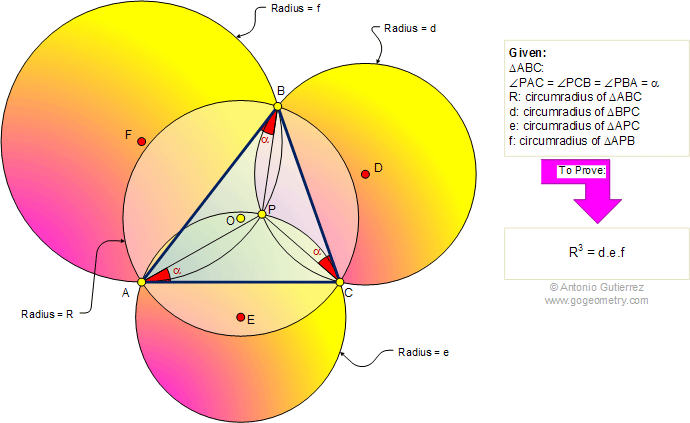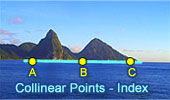# Geometry Problem 457: Triangle, First Brocard Point, Congruent angles, Circle, Circumradius, Common Chord.

 The figure shows a triangle ABC with circumradius R. The angles PAC, PCB, and PBA are congruent. If d, e, and f are the circumradii of triangles BPC, APC, and APB, respectively, prove that R3 = d.e.f. Point P is called the first Brocard point of the triangle ABC. The circumradius is the radius of the circumcircle. The circumcircle of a triangle is a circle which passes through all the vertices of the triangle.More Problems: Proposed Problem 458. Square, Semicircle, Circular Sector, Internal Common Tangent, Measurement. Proposed Problem 456. Three tangent circles, Center, Chord, Secant, Collinear. Proposed Problem 455. Rhombus, Inscribed Circle, Angle, Chord, 45 Degrees.Home | Search | Geometry | Problems | Art | All Problems | Visual Index | 451-460 | Circumcenter | Common Chord | Email | Post a comment | by Antonio Gutierrez